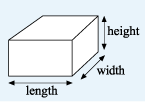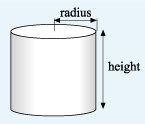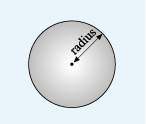Science, Maths & Technology

### Become an OU studentSucceed with maths: part 2

Start this free course now. Just create an account and sign in. Enrol and complete the course for a free statement of participation or digital badge if available.

# 4.1 Volume formulas

You may have already worked out that the formula for the volume of a cuboid can be calculated using the formula shown below.Figure 29 A cuboid with the length, width and height marked

Here are the formulas of various other shapes that you may encounter as well. Remember to make a note of them somewhere, as you may need one or another for the quizzes!

## Volume of cylinderFigure 30 A cylinder with the radius of the base and the height marked

## Volume of a sphereFigure 31 A sphere with the radius marked

Use the relevant formulas to complete this next activity and apply what you have learned in this section.

## Activity 8 A fuel tanker

Timing: Allow approximately 5 minutes
• a.The container on a fuel tanker is approximately cylindrical, with an internal diameter of about 2.25 m and an internal length of 10 m. Roughly how many cubic metres of fuel will it hold?

• a.

The length is 10 m, which is in this case equivalent to the height.

Substituting these values into the formula for the volume of a cylinder:

Rounding to the nearest cubic metre, the volume is 40 m3.

• b.The petrol station has an underground tank that measures 2.5 m by 4 m by 3 m. If the tank is empty, will it hold all the fuel in the tanker?